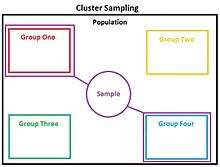# Cluster samplingCluster Sampling

Cluster sampling is a sampling technique used when "natural" but relatively heterogeneous groupings are evident in a statistical population. It is often used in marketing research. In this technique, the total population is divided into these groups (or clusters) and a simple random sample of the groups is selected. The elements in each cluster are then sampled. If all elements in each sampled cluster are sampled, then this is referred to as a "one-stage" cluster design. If a simple random subsample of elements is selected within each of these groups, this is referred to as a "two-stage" design. A common motivation for cluster sampling is to reduce the total number of interviews and costs given the desired accuracy. Assuming a fixed sample size, the technique gives more accurate results when most of the variation in the population is within the groups, not between them.

## Cluster elements

The population within a cluster should ideally be as heterogeneous as possible, but there should be homogeneity between cluster means. Each cluster should be a small-scale representation of the total population. The clusters should be mutually exclusive and collectively exhaustive. A random sampling technique is then used on any relevant clusters to choose which clusters to include in the study. In single-stage cluster sampling, all the elements from each of the selected clusters are used. In two-stage cluster sampling, a random sampling technique is applied to the elements from each of the selected clusters.

The main difference between cluster sampling and stratified sampling is that in cluster sampling the cluster is treated as the sampling unit so analysis is done on a population of clusters (at least in the first stage). In stratified sampling, the analysis is done on elements within strata. In stratified sampling, a random sample is drawn from each of the strata, whereas in cluster sampling only the selected clusters are studied. The main objective of cluster sampling is to reduce costs by increasing sampling efficiency. This contrasts with stratified sampling where the main objective is to increase precision.

There also exists multistage sampling, here more than two steps are taken in selecting clusters from clusters.

## Aspects of cluster sampling

One version of cluster sampling is area sampling or geographical cluster sampling. Clusters consist of geographical areas. Because a geographically dispersed population can be expensive to survey, greater economy than simple random sampling can be achieved by treating several respondents within a local area as a cluster. It is usually necessary to increase the total sample size to achieve equivalent precision in the estimators, but cost savings may make that feasible.

In some situations, cluster analysis is only appropriate when the clusters are approximately the same size. This can be achieved by combining clusters. If this is not possible, probability proportionate to size sampling is used. In this method, the probability of selecting any cluster varies with the size of the cluster, giving larger clusters a greater probability of selection and smaller clusters a lower probability. However, if clusters are selected with probability proportionate to size, the same number of interviews should be carried out in each sampled cluster so that each unit sampled has the same probability of selection.

Cluster sampling is used to estimate high mortalities in cases such as wars, famines and natural disasters.

• Can be cheaper than other methods – e.g. fewer travel expenses, administration costs.
• Feasibility: This method takes large populations into account. Since these groups are so large, deploying any other technique would be very difficult task. It is feasible only when you are dealing with large population.
• Economy: The regular two major concerns of expenditure, i.e., traveling and listing, are greatly reduced in this method. For example: Compiling research information about every house hold in city would be a very difficult, whereas compiling information about various blocks of the city will be easier. Here, traveling as well as listing efforts will be greatly reduced.
• Reduced variability: When estimates are being considered by any other method, reduced variability in results are observed. This may not be an ideal situation every time.

• Higher sampling error, which can be expressed in the so-called "design effect", the ratio between the number of subjects in the cluster study and the number of subjects in an equally reliable, randomly sampled unclustered study.
• Biased samples: If the group in population that is chosen as a sample has a biased opinion, then the entire population is inferred to have the same opinion. This may not be the actual case.

Errors: The other probabilistic methods give fewer errors than this method. For this reason, it is discouraged for beginners.

## More on cluster sampling

### Two-stage cluster sampling

Two-stage cluster sampling, a simple case of multistage sampling, is obtained by selecting cluster samples in the first stage and then selecting sample of elements from every sampled cluster. Consider a population of N clusters in total. In the first stage, n clusters are selected using ordinary cluster sampling method. In the second stage, simple random sampling is usually used. It is used separately in every cluster and the numbers of elements selected from different clusters are not necessarily equal. The total number of clusters N, number of clusters selected n, and numbers of elements from selected clusters need to be pre-determined by the survey designer. Two-stage cluster sampling aims at minimizing survey costs and at the same time controlling the uncertainty related to estimates of interest. This method can be used in health and social sciences. For instance, researchers used two-stage cluster sampling to generate a representative sample of the Iraqi population to conduct mortality surveys. Sampling in this method can be quicker and more reliable than other methods, which is why this method is now used frequently.